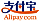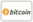Bookcover of Biased Estimators for the parameters of Linear Regression model
Booktitle:

# Biased Estimators for the parameters of Linear Regression model

LAP LAMBERT Academic Publishing (2013-05-08 )eligible for voucher
ISBN-13:

### 978-3-659-38873-6

ISBN-10:
3659388734
EAN:
9783659388736
Book language:
English
Blurb/Shorttext:
This work deal with biased estimation methods for estimating the parameters of general linear regression model when the data are ill-conditioned. We focus our attention on ordinary and generalized ridge regression estimators, Jackknife ridge estimators and principal components estimators. In chapter one introduction and historical review In chapter two basic concepts, definitions on linear regression model are presented. Moreover, the statistical properties of the ordinary least squares estimators are presented. Classes of biased estimators are discussed in chapter three when the data suffer from the multicollinearity problem. The procedures discussed in the preceding chapters were applied in chapter four to perform the regression analysis employing the data obtained from Midland Refineries Company in Iraq, for 12 years period in order to determine the effect of six different factors on the productivity of labor. The statistical programs, SPSS, and Minitab were employed to perform the required calculations.
Publishing house:
Website:
https://www.lap-publishing.com/
By (author) :
Bushra Abdalrasool Ali
Number of pages:
96
Published on:
2013-05-08
Stock:
Available
Category:
Mathematics
Price:
54.90 €
Keywords:
Singular Value Decomposition, Shrinkage Estimators, Ordinary ridge regression, Generalized ridge regression, Principal Component Estimators, Jackknife Technique### Categories